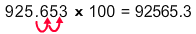# Multiply a Decimal by Powers of 10

Rate 0 stars Common Core
Quiz size:
Message preview:
Someone you know has shared Multiply a Decimal by Powers of 10 quiz with you:

To play this quiz, click on the link below:

https://www.turtlediary.com/quiz/multiply-a-decimal-by-powers-of-10.html

Hope you have a good experience with this site and recommend to your friends too.

Login to rate activities and track progress.
Login to rate activities and track progress.

When multiplying by a power of ten, move the decimal point to the right by as many places as there are 0s in the power of 10.

For example: Let's multiply 925.653 × 100.

To find the product, move the decimal point to the right by as many places as there are 0s in the power of 10.

925.653 × 100 = ?

Notice that there are two zeros in 100.

So, move the decimal point two places to the right.So, 925.653 × 100 = 92565.3

ds

A B C D E F G H I J K L M N O P Q R S T U V W X Y Z

### Help

##### Remember :

The smallest number is the one that comes first while counting.

##### Solution :

To arrange the given numbers in order from smallest to greatest, find the smallest number among all the given numbers.

21,27,23

21 is the smallest number.